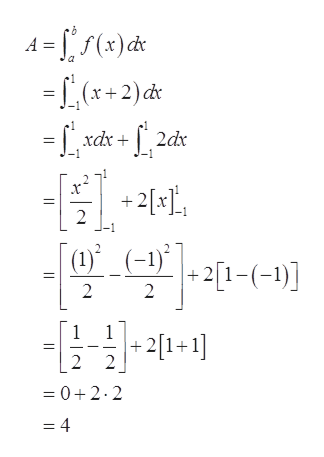# Find the area of the region between the graph of y=x+2, above x-axis, and between the lines x= -1 and x= 1

Question
4 views

Find the area of the region between the graph of y=x+2, above x-axis, and between the lines x= -1 and x= 1

check_circle

Step 1

Given:

Step 2

Graph:

Step 3

Find the ar...help_outlineImage Transcriptionclose15(x)c* =L (x+2)đt = [ , xdx + f ,2dx 1-1 +2[x], 2 L. (1)° _(-1)* + 2[1-(-1)] + 2[1+1] = 0+2.2 = 4 fullscreen

### Want to see the full answer?

See Solution

#### Want to see this answer and more?

Solutions are written by subject experts who are available 24/7. Questions are typically answered within 1 hour.*

See Solution
*Response times may vary by subject and question.
Tagged in

### Integration